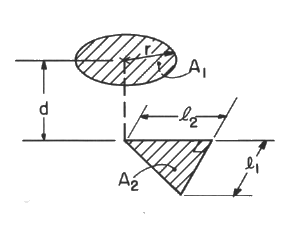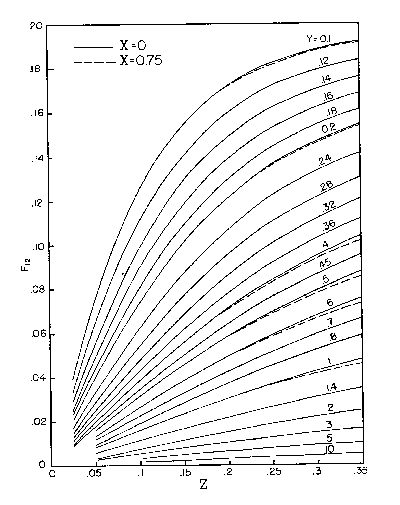# SECTION C Factors From Finite Areas to Finite Areas

## C-39: Circular disk to parallel right triangle; normal from center of circle passes through one acute vertex.Definitions: X=r/d; Y=d/l1; Z=d/l2
Graphical Results (for all X, Y; Z < 0.35):For X = 0, click HERE

For X = 0.25, click HERE

For X = 0.5, click HERE

For X = 0.75, click HERE